# How to Calculate and Solve for Gas Formation Volume Factor (FVF) | The Calculator Encyclopedia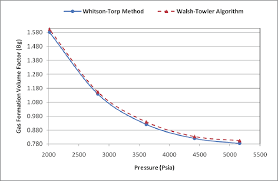The image above represents the gas FVF.

To compute for the gas FVF, three essential parameters are needed and these parameters are Z-Factor (Z), Temperature (T) and Pressure (P).

The formula for calculating the gas FVF:

Bg = 0.00504[ZT / P]

Where;

Bg = Gas FVF
Z = Z-Factor
T = (°R) Temperature
P = Pressure

Let’s solve an example;
Find the Gas FVF when the Z-Factor is 12, temperature is 30 and the pressure is 120.

This implies that;

Z = Z-Factor = 12
T = (°R) Temperature = 30
P = Pressure = 120

Bg = 0.00504[ZT / P]
Bg = 0.00504[12 x 30 / 120]
Bg = 0.00504[360 / 120]
Bg = 0.00504
Bg = 0.01512

Therefore, the gas FVF is 0.01512 bbl / scf.

Calculating for the Z-Factor when the Gas FVF, Temperature and Pressure is Given.

Z = Bg.P / 0.00504T

Where;

Z = Z-Factor
Bg = Gas FVF
T = (°R) Temperature
P = Pressure

Let’s solve an example;
Find the Z-Factor when the Gas FVF is 22, temperature is 16 and the pressure is 80.

This implies that;

Bg = Gas FVF = 22
T = (°R) Temperature = 16
P = Pressure = 80

Z = Bg.P / 0.00504T
Z = 22 x 80 / 0.00504 x 16
Z = 1760 / 0.08064
Z = 21825.39

Therefore, the Z-Factor is 21825.39.

Calculating for the Temperature when the Gas FVF, Z-Factor and the Pressure is Given.

T = Bg.P / 0.00504Z

Where;

T = (°R) Temperature
Z = Z-Factor
Bg = Gas FVF
P = Pressure

Let’s solve an example;
Find the Temperature when the Gas FVF is 44, Z-Factor is 29 and the pressure is 70.

This implies that;

Z = Z-Factor = 29
Bg = Gas FVF = 44
P = Pressure = 70

T = Bg.P / 0.00504Z
T = 44 x 70 / 0.00504 x 29
T = 3080 / 0.14616
T = 21072.79

Therefore, the temperature is 21072.79.

Calculating for the pressure when the Gas FVF, Z-Factor and the Temperature is Given.

P = 0.00504 (ZT) / Bg

Where;

P = Pressure
Bg = Gas FVF
T = (°R) Temperature
Z = Z-Factor

Let’s solve an example;
Find the pressure when the Gas FVF is 52, Z-Factor is 32 and the Temperature is 90.

This implies that;

Bg = Gas FVF = 52
T = (°R) Temperature = 90
Z = Z-Factor = 32

P = 0.00504 (ZT) / Bg
P = 0.00504 (32 x 90) / 52
P = 0.00504 (2880) / 52
P = 14.5152 / 52
P = 0.279

Therefore, the pressure is 0.279.

Nickzom Calculator – The Calculator Encyclopedia is capable of calculating the gas FVF.

To get the answer and workings of the gas FVF using the Nickzom Calculator – The Calculator Encyclopedia. First, you need to obtain the app.

You can get this app via any of these means:

To get access to the professional version via web, you need to register and subscribe for NGN 1,500 per annum to have utter access to all functionalities.
You can also try the demo version via https://www.nickzom.org/calculator

Apple (Paid) – https://itunes.apple.com/us/app/nickzom-calculator/id1331162702?mt=8
Once, you have obtained the calculator encyclopedia app, proceed to the Calculator Map, then click on Petroleum under EngineeringNow, Click on Fluid Properties under PetroleumNow, Click on Gas FVF under Fluid PropertiesThe screenshot below displays the page or activity to enter your values, to get the answer for the gas FVF according to the respective parameters which are the Z-Factor (Z), Temperature (T) and Pressure (P).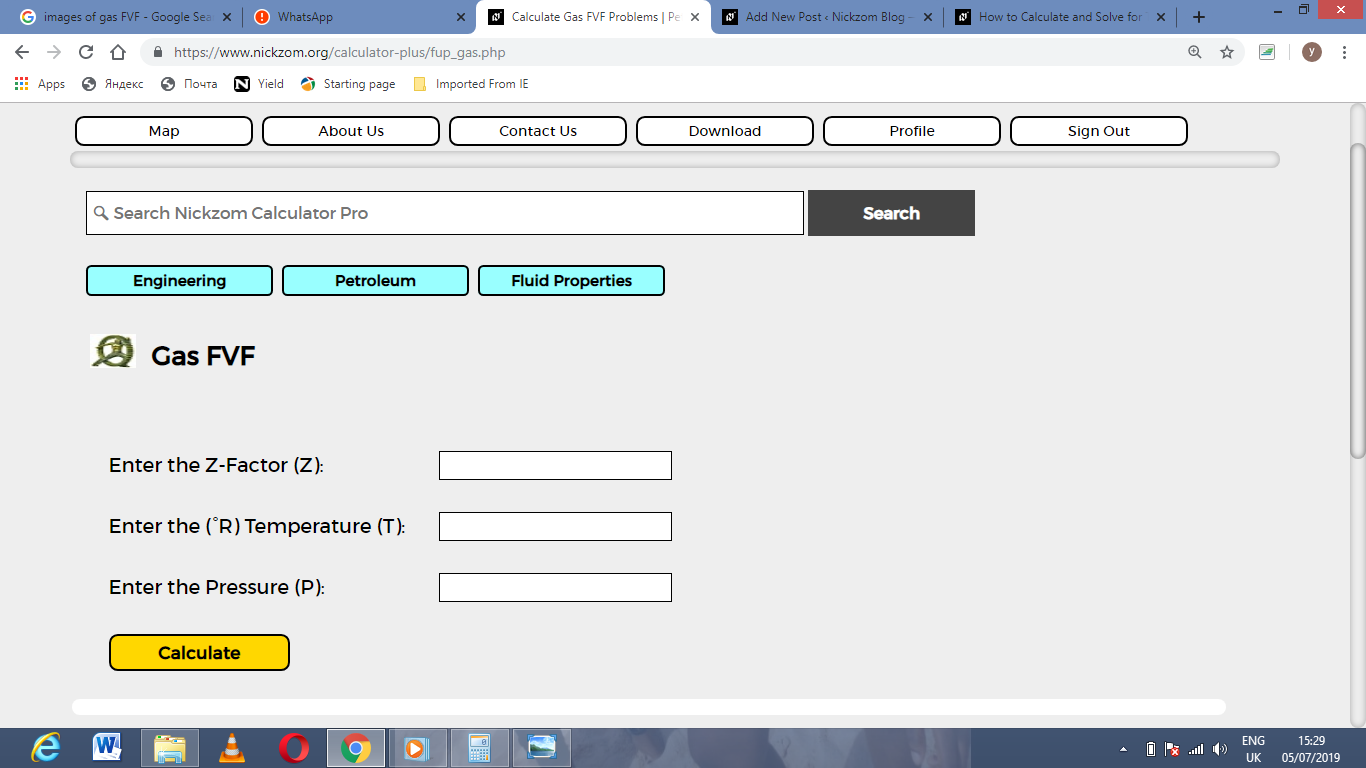Now, enter the values appropriately and accordingly for the parameters as required by the Z-Factor (Z) is 12, Temperature (T) is 30 and Pressure (P) is 120.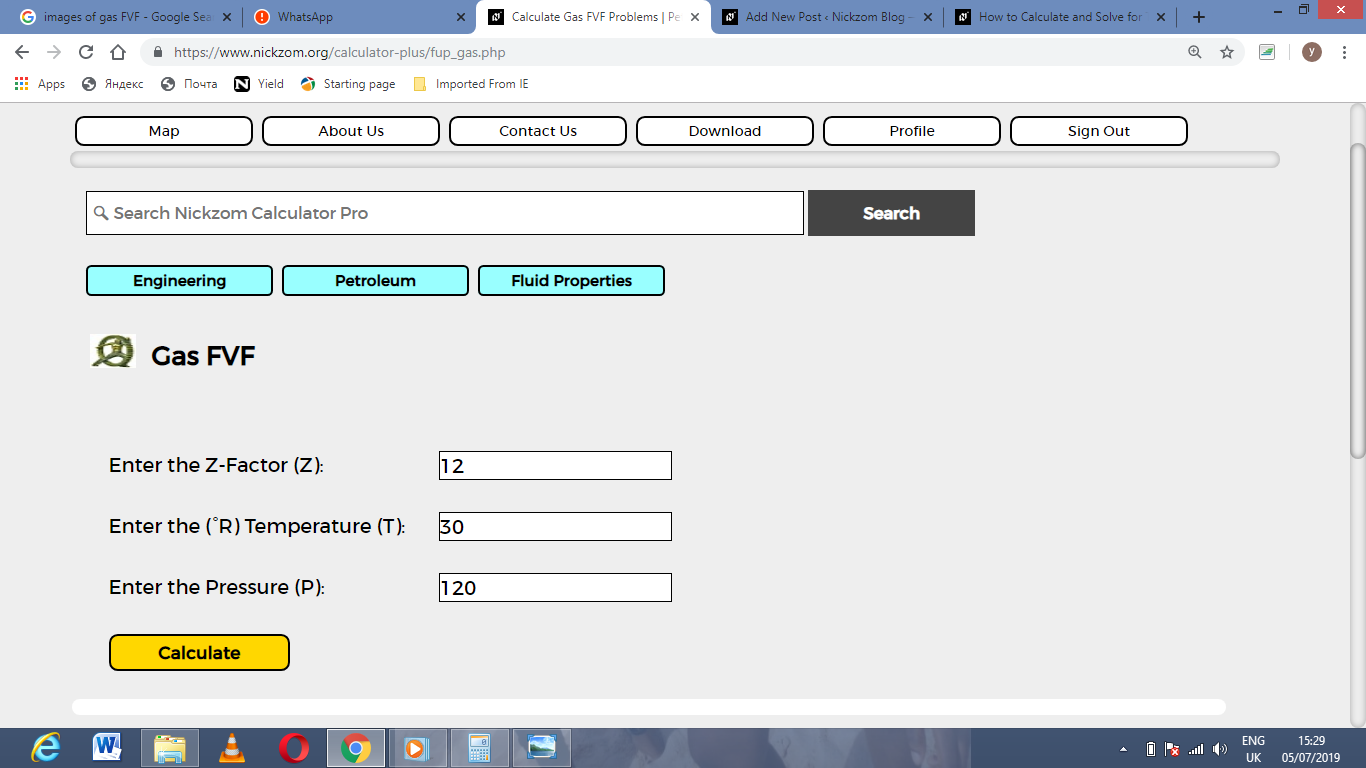Finally, Click on Calculate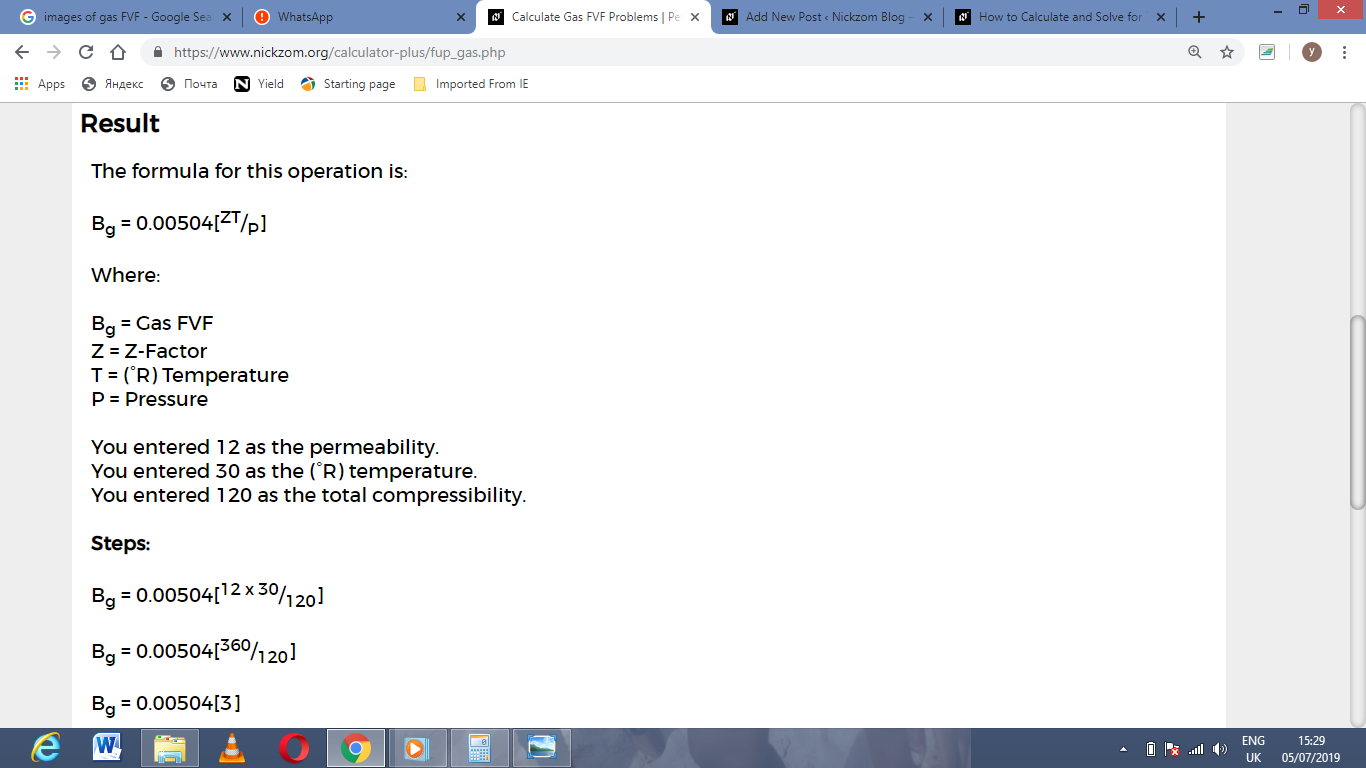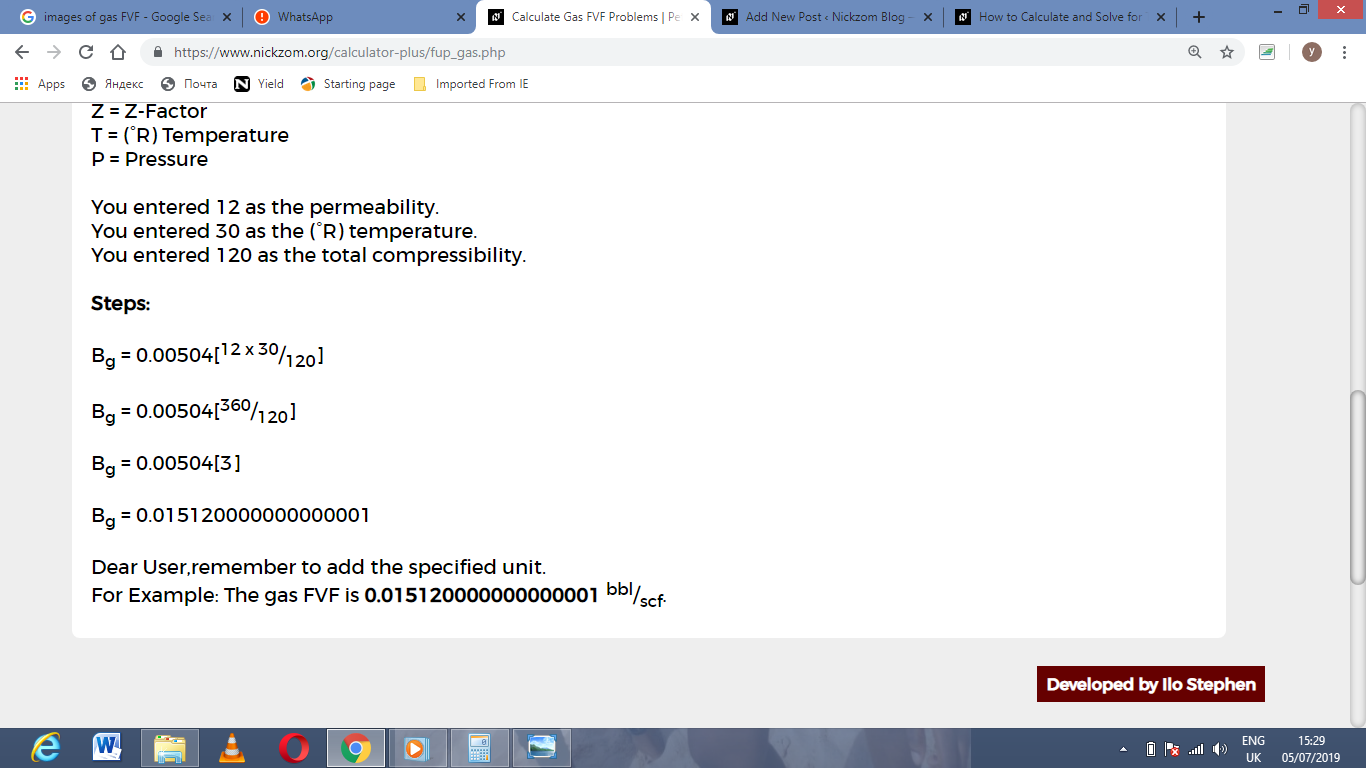As you can see from the screenshot above, Nickzom Calculator– The Calculator Encyclopedia solves for the gas FVF and presents the formula, workings and steps too.